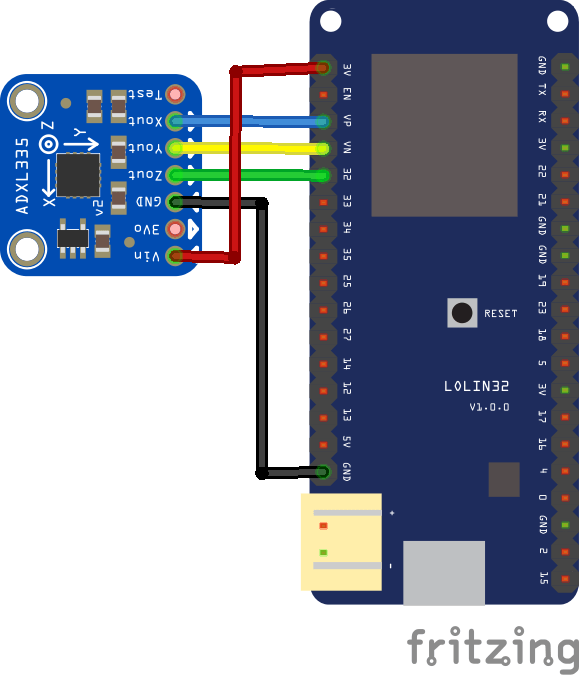Home » ESP32 and ADXL335 accelerometer example

# ESP32 and ADXL335 accelerometer example

The ADXL335 is a small, thin, low power, complete 3-axis accelerometer with signal conditioned voltage outputs. The product measures acceleration with a minimum full-scale range of ±3 g. It can measure the static acceleration of gravity in tiltsensing applications, as well as dynamic acceleration resulting from motion, shock, or vibration.

The user selects the bandwidth of the accelerometer using the CX, CY, and CZ capacitors at the XOUT, YOUT, and ZOUT pins. Bandwidths can be selected to suit the application, with a range of 0.5 Hz to 1600 Hz for X and Y axes, and a range of 0.5 Hz to 550 Hz for the Z axis.

Here is a typical module that makes it easy to work with the ADXL335

Features:

Name: ADXL335 module (triaxial accelerometer analog output)
Model: GY-61
Power supply :3-5v
Analog X, Y, Z three-axis output

Schematic

The following layout shows how to wire the ADXL335 module up to an ESP32 (LOLIN32)Code

```const int xpin = A0; // x-axis of the accelerometer
const int ypin = A3; // y-axis
const int zpin = A4; // z-axis

void setup()
{
Serial.begin(9600);
}

void loop()
{
delay(1); //
delay(1);

float zero_G = 512.0; //ADC is 0~1023 the zero g output equal to Vs/2
float scale = 102.3; //ADXL335330 Sensitivity is 330mv/g
//330 * 1024/3.3/1000
Serial.print(((float)x - 331.5)/65*9.8); //print x value on serial monitor
Serial.print("\t");
Serial.print(((float)y - 329.5)/68.5*9.8); //print y value on serial monitor
Serial.print("\t");
Serial.print(((float)z - 340)/68*9.8); //print z value on serial monitor
Serial.print("\n");
delay(1000); //wait for 1 second
}

```

Output

Open the serial monitor and you should see something like this

281.56 190.49 227.99
282.16 188.63 227.56
241.91 172.47 268.92
285.33 230.55 247.74
234.97 260.45 197.73
209.04 263.74 249.76
214.62 150.43 242.41
322.57 165.74 211.56
264.98 170.75 222.09
260.76 168.17 216.32